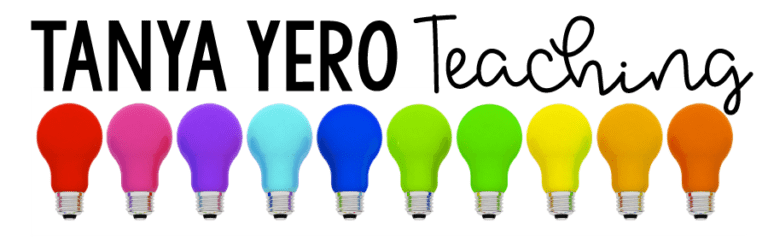# 1st Grade Word Problems Morning Work | YEARLONG | Guided Math Spiral Review

\$13.00

Total Pages: 120
File Size: 46 MB

## Description

Visual representation is important to students. Get your students excited about math by Using Snapshots of Math Word Problems!

This purchase contains word problems for all the 1st grade math standards. Each word problem is applicable for young learners and includes a photo for visual representation. Each question is on a PowerPoint slide for easy use and can be used during a whole group lesson, to introduce a skill, or extra practice.

**There are 5 questions for each standard.**

Standards and Topics Covered

Operations and Algebraic Thinking
➥ 1.OA.1 – Use addition and subtraction within 20 to solve word problems
➥ 1.OA.2 – Solve word problems that call for addition of three whole numbers whose sum is less than or equal to 20
➥ 1.OA.3 – Apply properties of operations as strategies to add and subtract
➥ 1.OA.4 – Understand subtraction as an unknown-addend problem
➥ 1.OA.5 – Relate counting to addition and subtraction
➥ 1.OA.6 – Add and subtract within 20, demonstrating fluency for addition and subtraction within 10
➥ 1.OA.7 – Understand the meaning of the equal sign
➥ 1.OA.8 – Determine the unknown whole number in an addition or subtraction equation

Number and Operations in Base Ten
➥ 1.NBT.1 – Count to 120, starting at any number less than 120
➥ 1.NBT.2 – Understand that the two digits of a two-digit number represent amounts of tens and ones
➥ 1.NBT.3 – Compare two two-digit numbers
➥ 1.NBT.4 – Add within 100, including adding a two-digit number and a one-digit number
➥ 1.NBT.5 – Given a two-digit number, mentally find 10 more or 10 less than the number
➥ 1.NBT.6 – Subtract multiples of 10 in the range 10-90 from multiples of 10 in the range 10-90

Measurement and Data
➥ 1.MD.1 – Order objects by length
➥ 1.MD.2 – Express the length of an object as a whole number of length units, by laying multiple copies of a shorter object
➥ 1.MD.3 – Tell and write time in hours and half-hours using analog and digital clocks
➥ 1.MD.4 – Organize, represent, and interpret data with up to three categories

Geometry
➥ 1.G.1 – Identifying attributes of shapes
➥ 1.G.2 – Composing shapes
➥ 1.G.3 – Partition circles and rectangles into equal shares